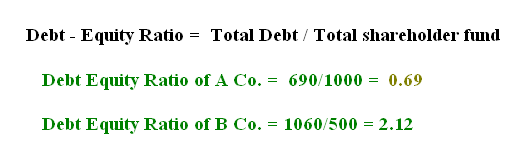222

## Latest \$type=blogging\$count=5\$author=hide\$comment=hide\$label=hide\$date=hide\$show=home

Financial analysis converts raw information of financial statements in useful financial information. Only after financial analysis, we can use financial statements for decision making. These financial information are useful for planning. For example, we can estimate our future ability of earning on advertising if we did financial analysis of our advertising expenses with direct return on the investment in advertising. Like this, we can do financial analysis of each and every item of profit and loss account, balance sheet and cash flow statement.

For doing financial analysis, we use following tools.

1. Tool of Financial Analysis : Financial Statement Analysis

In financial statement analysis is that tool of financial analysis in which we create and highlight the significant relationships of different items of financial statement. We also interpret that relationship in simple words. For example, an investor may be interested to know past earning data with its relationship with company's investments. Two type of financial statement analysis are very important. One is horizontal analysis and second is vertical analysis. In horizontal analysis, we compare all items of balance sheet and profit and loss account with previous years' balance sheet and profit and loss account's items. In vertical analysis, we convert each element of the information into a percentage of the total amount of statement so as to establish relationship with other components of the same statement.

2. Tool of Financial Analysis : Ratio Analysis

Actually, it is also financial statement analysis but for detail study we can make separate topic of study. We calculate different ratios like revenue ratios, balance sheet ratios and mixed ratios. Ratio analysis is based on fact, if we create relationship between two accounting figures, we can get useful information relating to performance, strengths and weakness. For example, we know that according to rule of thumb, debt equity ratio should not more than 2:1 because it is risky. As an investor, we calculate debt -equity ratio before invest our money in the form of debt. Here are two company and we have calculated its debt equity ratio.

We see the debt equity ratio of B Co. is more than our rule of thumb. So, we should invest in company A's debentures.: 1
1.In comment, you can give your feedback, reviews, ideas for improving content or ask question relating to written content.

Name

ltr
item
Accounting Education: Introduction of Financial Analysis
Introduction of Financial Analysis
http://4.bp.blogspot.com/-pbNGJUmyQdc/TkjWvjCJ3hI/AAAAAAAAGzI/0UZurmnIlp8/s1600/debt+equity+ratio.PNG
http://4.bp.blogspot.com/-pbNGJUmyQdc/TkjWvjCJ3hI/AAAAAAAAGzI/0UZurmnIlp8/s72-c/debt+equity+ratio.PNG
Accounting Education
https://www.svtuition.org/2011/08/introduction-of-financial-analysis.html
https://www.svtuition.org/
https://www.svtuition.org/
https://www.svtuition.org/2011/08/introduction-of-financial-analysis.html
true
2410664366776677676
UTF-8